# coseq package: Quick-start guide

#### 2019-05-02

coseq is a package to perform clustering analysis of sequencing data (e.g., co-expression analysis of RNA-seq data), using transformed profiles rather than the raw counts directly. The package implements two distinct strategies in conjunction with transformations: 1) MacQueen’s K-means algorithm and 2) Gaussian mixture models. For both strategies model selection is provided using the slope heuristics approach and integrated completed likelihood (ICL) criterion, respectively. Note that for backwards compatibility, coseq also provides a wrapper for the Poisson mixture model originally proposed in Rau et al. (2015) and implemented in the HTSCluster package.

The methods implemented in this package are described in detail in the following three publications.

• Godichon-Baggioni, A., Maugis-Rabusseau, C. and Rau, A. (2018) Clustering transformed compositional data using K-means, with applications in gene expression and bicycle sharing system data. Journal of Applied Statistics, doi:10.1080/02664763.2018.1454894. [Paper that introduced the use of the K-means algorithm for RNA-seq profiles after transformation via the centered log ratio (CLR) or log centered log ratio (logCLR) transformation.]

• Rau, A. and Maugis-Rabusseau, C. (2018) Transformation and model choice for co-expression analayis of RNA-seq data. Briefings in Bioinformatics, 19(3)-425-436. [Paper that introduced the idea of clustering profiles for RNA-seq co-expression, and suggested using Gaussian mixture models in conjunction with either the arcsine or logit transformation.]

• Rau, A., Maugis-Rabusseau, C., Martin-Magniette, M.-L., Celeux, G. (2015) Co-expression analysis of high-throughput transcriptome sequencing data with Poisson mixture models. Bioinformatics, 31(9): 1420-1427. link [Paper that introduced the use of Poisson mixture models for RNA-seq counts.]

Below, we provide a quick-start guide using a subset of RNA-seq data to illustrate the functionalities of the coseq package. In this document, we focus on the methods described in Rau and Maugis-Rabusseau (2018) and Godichon-Baggioni et al. (2018). For more information about the method described in Rau et al. (2015), see the HTSCluster vignette.

## Quick start (tl;dr)

A standard coseq analysis takes the following form, where counts represents a matrix or data.frame of gene-level counts arising from an RNA-seq experiment (of dimension n x d for n genes and d samples). Results, exported in the form of a coseqResults S4 object, can easily be examined using standard summary and plot functions (see below and the User’s Guide for examples of plots that may be obtained):

run <- coseq(counts, K=2:25)
summary(run)
plot(run)

The cluster labels for the selected model obtained from the coseq clustering may easily be obtained using the clusters function:

clusters(run)

Similarly, the conditional probabilities of cluster membership for each gene in the selected coseq clustering model may be obtained using the assay accessor function:

assay(run)

Note that unless otherwise specified by the user, coseq uses the following default parameters:

• K-means algorithm
• Log centered log transformation (logclr) on profiles
• Library size normalization via the Trimmed Mean of M-values (TMM) approach
• Model selection (choice of the number of clusters) via the slope heuristics approach
• No parallelization.

## Co-expression analysis with coseq

For the remainder of this vignette, we make use of the mouse neocortex RNA-seq data from Fietz et al. (2012) (available at https://perso.math.univ-toulouse.fr/maugis/mixstatseq/packages and as an ExpressionSet object called fietz in coseq). The aim in this study was to investigate the expansion of the neocortex in five embryonic (day 14.5) mice by analyzing the transcriptome of three regions: the ventricular zone (VZ), subventricular zone (SVZ) and cortical place (CP).

We begin by first loading the necessary R packages as well as the data.

library(coseq)
library(Biobase)
library(corrplot)

data("fietz")
counts <- exprs(fietz)
conds <- pData(fietz)$tissue ## Equivalent to the following: ## counts <- read.table("http://www.math.univ-toulouse.fr/~maugis/coseq/Fietz_mouse_counts.txt", ## header=TRUE) ## conds <- c(rep("CP",5),rep("SVZ",5),rep("VZ",5)) The coseq package fits either a Gaussian mixture model (Rau and Maugis-Rabusseau, 2018) or uses the K-means algorithm (Godichon-Baggioni et al., 2018) to cluster transformed normalized expression profiles of RNA-seq data. Normalized expression profiles correspond to the proportion of normalized reads observed for gene i with respect to the total observed for gene i across all samples: $p_{ij} = \frac{y_{ij}/s_{j} +1}{\sum_{j'} y_{ij'}/s_{j'} +1},$ where $$s_j$$ are normalization scaling factors (e.g., after applying TMM normalization to library sizes) and $$y_{ij}$$ represents the raw count for gene $$i$$ in sample $$j$$. ### Transformations for normalized profiles with the Gaussian mixture model Since the coordinates of $$\mathbf{p}_i$$ are linearly dependent (causing estimation problems for a Gaussian mixture distribution), weconsider either the arcsine or logit transformation of the normalized profiles $$p_{ij}$$: $g_{\text{arcsin}}(p_{ij}) = \text{arcsin}\left( \sqrt{ p_{ij} } \right) \in [0, \pi/2], \text{ and}$ $g_{\text{logit}}(p_{ij}) = \text{log}_2 \left( \frac{p_{ij}}{1-p_{ij}} \right) \in (-\infty, \infty).$ Then the distribution of the transformed normalized expression profiles is modeled by a general multidimensional Gaussian mixture $f(.|\theta_K) = \sum_{k=1}^K \pi_k \phi(.|\mu_k,\Sigma_k)$ where $$\theta_K=(\pi_1,\ldots,\pi_{K-1},\mu_1,\ldots,\mu_K,\Sigma_1,\ldots,\Sigma_K)$$, $$\pi=(\pi_1,\ldots,\pi_K)$$ are the mixing proportions and $$\phi(.|\mu_k,\Sigma_k)$$ is the $$q$$-dimensional Gaussian density function with mean $$\mu_k$$ and covariance matrix $$\Sigma_k$$. To estimate mixture parameters $$\theta_K$$ by computing the maximum likelihood estimate (MLE), an Expectation-Maximization (EM) algorithm is used via the Rmixmod package. Finally, let $$\hat t_{ik}$$ be the conditional probability that observation $$i$$ arises from the $$k$$th component of the mixture $$f(.|\hat \theta_{\hat K})$$. Each observation $$i$$ is assigned to the component maximizing the conditional probability $$\hat t_{ik}$$ i.e., using the so-called maximum a posteriori (MAP) rule. ### Transformations for normalized profiles with K-means For the K-means algorithm, we consider three separate transformations of the profiles $$p_{i}$$ that are well adapted to compositional data (see Godichon-Baggioni et al., 2017 for more details): • Identity (i.e., no transformation) • Centered log ratio (CLR) transformation • Log centered log ratio (logCLR) transformation Then, the aim is to minimize the sum of squared errors (SSE), defined for each set of clustering $$\mathcal{C}^{(K)}=\left( C_{1},...,C_{k}\right)$$ by $\begin{equation*} \text{SSE} \left( \mathcal{C}^{(K)}\right) := \sum_{k=1}^{K}\sum_{i \in C_{k}} \left\| h \left( y_{i}\right) - \mu_{k,h} \right\|_{2}^{2} , \end{equation*}$ with $$i \in C_{k}$$ if $$\left\| h\left( y_{i}\right) - \mu_{k,h} \right\|_{2} = \min_{k'=1,\ldots,K} \left\| y_{i}- \mu_{k',h} \right\|_{2}$$, and $\mu_{k,h}= \frac{1}{|C_{k} |}\sum_{i \in C_{k}}h \left( y_{i} \right),$ and $$h$$ is the chosen transformation. In order to minimize the SSE, we use the well-known MacQueen’s K-means algorithm. ### Model selection Because the number of clusters $$K$$ is not known a priori, we fit a collection of models (here $$K$$ = 2, …, 25) and use either the Integrated Completed Likelihood (ICL) criterion (in the case of the Gaussian mixture model) or the slope heuristics (in the case of the K-means algorithm) to select the best model in terms of fit, complexity, and cluster separation. ### Other options If desired, we can set a filtering cutoff on the mean normalized counts via the meanFilterCutoff argument to remove very weakly expressed genes from the co-expression analysis; in the interest of faster computation for this vignette, in this example we filter all genes with mean normalized expression less than 200 (for the Gaussian mixture model) or less than 50 (for the K-means algorithm). Note that if desired, parallel execution using BiocParallel can be specified via the parallel argument: run <- coseq(..., parallel=TRUE) ## Running coseq The collection of co-expression models for the logCLR-transformed profiles using the K-means algorithm may be obtained as follows (note that we artificially set the number of maximum allowed iterations and number of random starts to be low for faster computational time in this vignette): runLogCLR <- coseq(counts, K=2:25, transformation="logclr",norm="TMM", meanFilterCutoff=50, model="kmeans", nstart=1, iter.max=10) ## **************************************** ## coseq analysis: kmeans approach & logclr transformation ## K = 2 to 25 ## Use set.seed() prior to running coseq for reproducible results. ## **************************************** ## Running K = 2 ... ## Running K = 3 ... ## Running K = 4 ... ## Running K = 5 ... ## Running K = 6 ... ## Running K = 7 ... ## Running K = 8 ... ## Running K = 9 ... ## Running K = 10 ... ## Running K = 11 ... ## Running K = 12 ... ## Running K = 13 ... ## Running K = 14 ... ## Running K = 15 ... ## Running K = 16 ... ## Running K = 17 ... ## Running K = 18 ... ## Running K = 19 ... ## Running K = 20 ... ## Running K = 21 ... ## Running K = 22 ... ## Running K = 23 ... ## Running K = 24 ... ## Running K = 25 ... The collection of Gaussian mixture models for the arcsine-transformed and logit-transformed profiles may be obtained as follows (as before, we set the number of iterations to be quite low for computational speed in this vignette): runArcsin <- coseq(counts, K=2:20, model="Normal", transformation="arcsin", meanFilterCutoff=200, iter=10) ## **************************************** ## coseq analysis: Normal approach & arcsin transformation ## K = 2 to 20 ## Use set.seed() prior to running coseq for reproducible results. ## **************************************** ## Running K = 2 ... ## Running K = 3 ... ## Running K = 4 ... ## Running K = 5 ... ## Running K = 6 ... ## Running K = 7 ... ## Running K = 8 ... ## Running K = 9 ... ## Running K = 10 ... ## Running K = 11 ... ## Running K = 12 ... ## Running K = 13 ... ## Running K = 14 ... ## Running K = 15 ... ## Running K = 16 ... ## Running K = 17 ... ## Running K = 18 ... ## Running K = 19 ... ## Running K = 20 ... runLogit <- coseq(counts, K=2:20, model="Normal", transformation="logit", meanFilterCutoff=200, verbose=FALSE, iter=10) ## **************************************** ## coseq analysis: Normal approach & logit transformation ## K = 2 to 20 ## Use set.seed() prior to running coseq for reproducible results. ## **************************************** In all cases, the resulting output of a call to coseq is an S4 object of class coseqResults. class(runArcsin) ##  "coseqResults" ## attr(,"package") ##  "coseq" runArcsin ## An object of class coseqResults ## 4230 features by 15 samples. ## Models fit: K = 2 ... 20 ## Chosen clustering model: K = 9 To choose the most appropriate transformation to use (arcsine versus logit) in a Gaussian mixture model, we may use the corrected ICL, where the minimum value corresponds to the selected model. Note that this feature is not available for the K-means algorithm. compareICL(list(runArcsin, runLogit))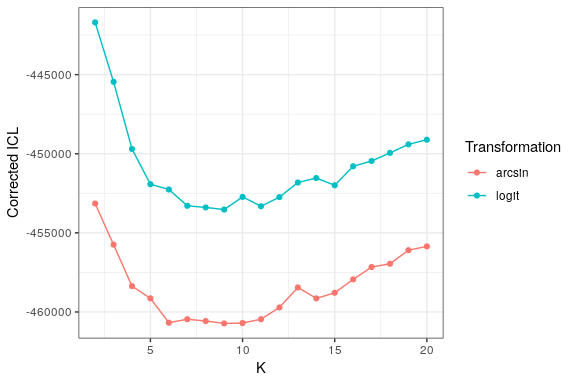This indicates that the preferred transformation is the arcsine, and the selected model has K = 9 clusters. We can additionally explore how similar the models with K = 8 to 12 are using the adjusted Rand index (ARI) via the compareARI function. Values close to 1 indicate perfect agreement, while values close to 0 indicate near random partitions. compareARI(runArcsin, K=8:12)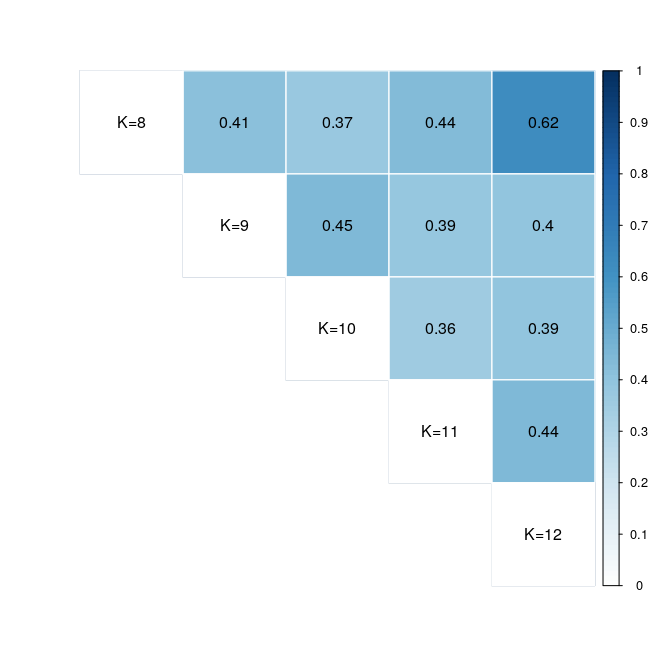## K=8 K=9 K=10 K=11 K=12 ## K=8 1 0.41 0.37 0.44 0.62 ## K=9 1 0.45 0.39 0.4 ## K=10 1 0.36 0.39 ## K=11 1 0.44 ## K=12 1 Note that because the results of coseq depend on the initialization point, results from one run to another may vary; as such, in practice, it is typically a good idea to re-estimate the same collection of models a few times (e.g., 5) to avoid problems linked to initialization. ## Exploring coseq results Results from a coseq analysis can be explored and summarized in a variety of ways. First, a call to the summary function provides the number of clusters selected for the ICL model selection approach, number of genes assigned to each cluster, and if desired the per-gene cluster means. summary(runArcsin) ## ************************************************* ## Model: Gaussian_pk_Lk_Ck ## Transformation: arcsin ## ************************************************* ## Clusters fit: 2,3,4,5,6,7,8,9,10,11,12,13,14,15,16,17,18,19,20 ## Clusters with errors: --- ## Selected number of clusters via ICL: 9 ## ICL of selected model: -365405.9 ## ************************************************* ## Number of clusters = 9 ## ICL = -365405.9 ## ************************************************* ## Cluster sizes: ## Cluster 1 Cluster 2 Cluster 3 Cluster 4 Cluster 5 Cluster 6 Cluster 7 ## 439 273 1141 975 587 38 415 ## Cluster 8 Cluster 9 ## 253 109 ## ## Number of observations with MAP > 0.90 (% of total): ## 3371 (79.69%) ## ## Number of observations with MAP > 0.90 per cluster (% of total per cluster): ## Cluster 1 Cluster 2 Cluster 3 Cluster 4 Cluster 5 Cluster 6 Cluster 7 ## 338 263 896 750 489 34 273 ## (76.99%) (96.34%) (78.53%) (76.92%) (83.3%) (89.47%) (65.78%) ## Cluster 8 Cluster 9 ## 226 102 ## (89.33%) (93.58%) Next, a variety of plots may be explored using the plot function: • logLike (log-likelihood plotted versus number of clusters), • ICL (ICL plotted versus number of clusters), • profiles(line plots of profiles in each cluster), • boxplots (boxplots of profiles in each cluster), • probapost_boxplots (boxplots of maximum conditional probabilities per cluster), • probapost_barplots (number of observations with a maximum conditional probability greater than a given threshold per cluster), • probapost_histogram (histogram of maximum conditional probabilities over all clusters). By default, all of these plots are produced simultaneously unless specific graphics are requested via the graphs argument. In addition, a variety of options are available to specify the number of graphs per row/column in a grid layout, whether profiles should be averaged or summed over conditions (collapse_reps), whether condition labels should be used, whether clusters should be ordered according to their similarity, etc. Note that the histogram of maximum conditional probabilities of cluster membership for all genes (probapost_histogram), and boxplots and barplots of maximum conditional probabilities of cluster membership for the genes assigned to each cluster (probapost_boxplots, probapost_barplots) help to evaluate the degree of certitude accorded by the model in assigning genes to clusters, as well as whether some clusters are attribued a greater degree of uncertainty than others. ## To obtain all plots ## plot(runArcsin) plot(runArcsin, graphs="boxplots") ##$boxplots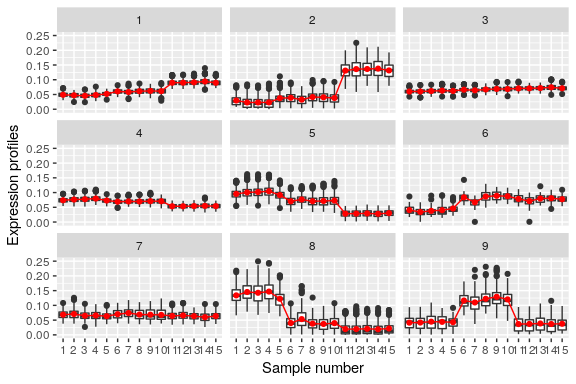plot(runArcsin, graphs="boxplots", conds=conds)
## $boxplots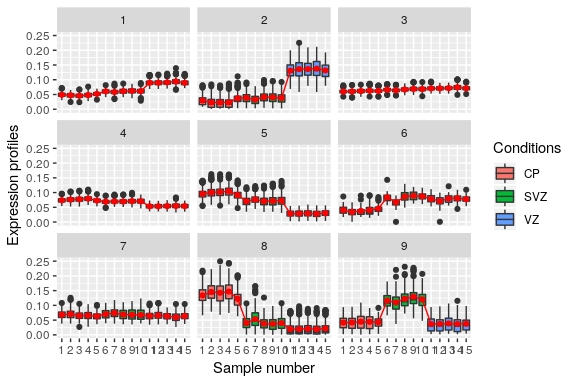plot(runArcsin, graphs="boxplots", conds=conds, collapse_reps = "sum") ##$boxplots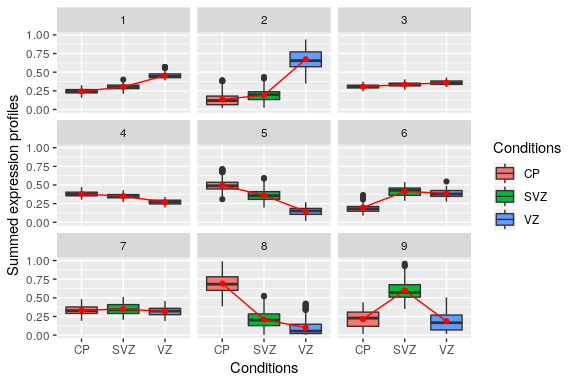plot(runArcsin, graphs="profiles")
## $profiles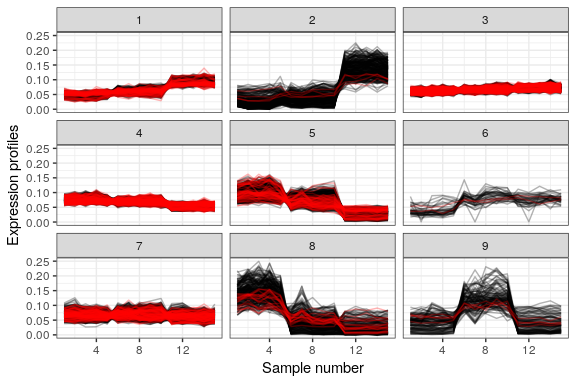plot(runArcsin, graphs="probapost_boxplots") ##$probapost_boxplots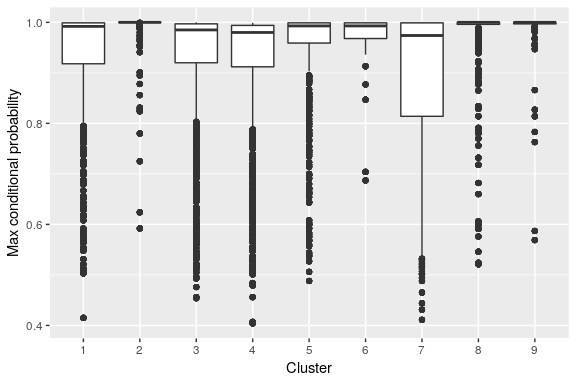plot(runArcsin, graphs="probapost_histogram")
## $probapost_histogram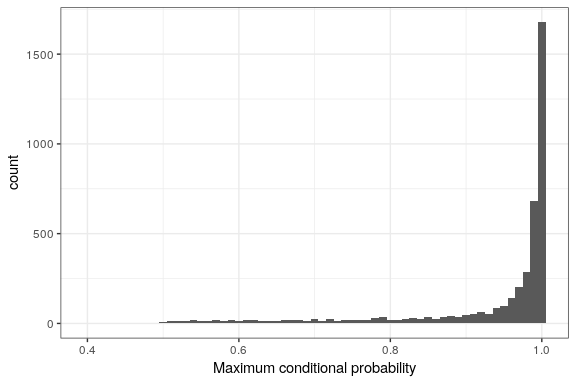If desired the per-cluster correlation matrices estimated in the Gaussian mixture model may be obtained by calling NormMixParam. These matrices may easily be visualized using the corrplot package. rho <- NormMixParam(runArcsin)$rho
## Covariance matrix for cluster 1
rho1 <- rho[,,1]
colnames(rho1) <- rownames(rho1) <- paste0(colnames(rho1), "\n", conds)
corrplot(rho1)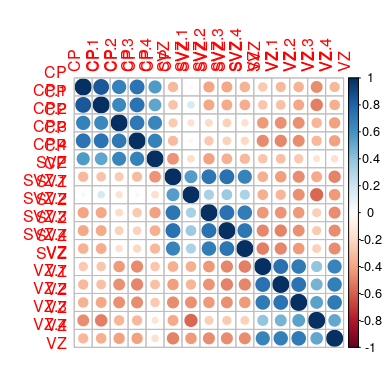Finally, cluster labels and conditional probabilities of cluster membership (as well as a variety of other information) are easily accessible via a set of accessor functions.

labels <- clusters(runArcsin)
table(labels)
## labels
##    1    2    3    4    5    6    7    8    9
##  439  273 1141  975  587   38  415  253  109
probapost <- assay(runArcsin)
head(probapost)
##        Cluster_1 Cluster_2 Cluster_3 Cluster_4 Cluster_5 Cluster_6
## Gene1      0.001     0.000     0.998     0.000     0.000         0
## Gene4      0.000     0.000     0.000     0.995     0.004         0
## Gene10     0.000     0.000     0.998     0.002     0.000         0
## Gene14     0.000     0.000     0.974     0.016     0.000         0
## Gene16     0.055     0.000     0.941     0.000     0.000         0
## Gene17     0.002     0.998     0.000     0.000     0.000         0
##        Cluster_7 Cluster_8 Cluster_9
## Gene1      0.001         0     0.000
## Gene4      0.001         0     0.000
## Gene10     0.000         0     0.000
## Gene14     0.011         0     0.000
## Gene16     0.003         0     0.000
## Gene17     0.000         0     0.001
metadata(runArcsin)
## $nbCluster ## K=2 K=3 K=4 K=5 K=6 K=7 K=8 K=9 K=10 K=11 K=12 K=13 K=14 K=15 K=16 ## 2 3 4 5 6 7 8 9 10 11 12 13 14 15 16 ## K=17 K=18 K=19 K=20 ## 17 18 19 20 ## ##$logLike
##      K=2      K=3      K=4      K=5      K=6      K=7      K=8      K=9
## 180249.4 182147.5 184152.4 185305.4 186649.0 187380.6 187875.6 188554.9
##     K=10     K=11     K=12     K=13     K=14     K=15     K=16     K=17
## 189261.1 189705.7 189900.5 189877.5 190854.3 191156.3 191229.0 191527.1
##     K=18     K=19     K=20
## 191816.8 192180.8 192516.7
##
## $ICL ## K=2 K=3 K=4 K=5 K=6 K=7 K=8 ## -357826.9 -360429.0 -363050.9 -363821.0 -365360.7 -365140.9 -365255.5 ## K=9 K=10 K=11 K=12 K=13 K=14 K=15 ## -365405.9 -365381.8 -365140.3 -364396.2 -363132.3 -363824.3 -363469.3 ## K=16 K=17 K=18 K=19 K=20 ## -362622.6 -361843.8 -361635.5 -360777.9 -360537.7 ## ##$nbClusterError
## integer(0)
##
## $GaussianModel ##  "Gaussian_pk_Lk_Ck" likelihood(runArcsin) ## K=2 K=3 K=4 K=5 K=6 K=7 K=8 K=9 ## 180249.4 182147.5 184152.4 185305.4 186649.0 187380.6 187875.6 188554.9 ## K=10 K=11 K=12 K=13 K=14 K=15 K=16 K=17 ## 189261.1 189705.7 189900.5 189877.5 190854.3 191156.3 191229.0 191527.1 ## K=18 K=19 K=20 ## 191816.8 192180.8 192516.7 nbCluster(runArcsin) ## K=2 K=3 K=4 K=5 K=6 K=7 K=8 K=9 K=10 K=11 K=12 K=13 K=14 K=15 K=16 ## 2 3 4 5 6 7 8 9 10 11 12 13 14 15 16 ## K=17 K=18 K=19 K=20 ## 17 18 19 20 ICL(runArcsin) ## K=2 K=3 K=4 K=5 K=6 K=7 K=8 ## -357826.9 -360429.0 -363050.9 -363821.0 -365360.7 -365140.9 -365255.5 ## K=9 K=10 K=11 K=12 K=13 K=14 K=15 ## -365405.9 -365381.8 -365140.3 -364396.2 -363132.3 -363824.3 -363469.3 ## K=16 K=17 K=18 K=19 K=20 ## -362622.6 -361843.8 -361635.5 -360777.9 -360537.7 model(runArcsin) ##  "Normal" transformationType(runArcsin) ##  "arcsin" The data used to fit the mixture model (transformed normalized profiles) as well as the normalized profiles themselves are stored as DataFrame objects that may be accessed with the corresponding functions: ## arcsine-transformed normalized profiles tcounts(runArcsin) ## DataFrame with 4230 rows and 15 columns ## CP CP.1 CP.2 ## <numeric> <numeric> <numeric> ## Gene1 0.236598173091045 0.228104752321001 0.241288674880122 ## Gene4 0.284826062120736 0.293176655713238 0.311890705392502 ## Gene10 0.253392009568601 0.263879866929211 0.253059933405496 ## Gene14 0.249871003807869 0.241841028766843 0.253633086159366 ## Gene16 0.230002856738101 0.235796495087209 0.249497115867557 ## ... ... ... ... ## Gene8946 0.107453539656086 0.0319221126659155 0.0502666347355386 ## Gene8948 0.222996022383283 0.218111459621408 0.248181591083828 ## Gene8950 0.23043323831067 0.219640579402256 0.266896858705074 ## Gene8951 0.168093297721759 0.132508885394036 0.152362137940985 ## Gene8952 0.323130913168413 0.323515106720572 0.365093451318071 ## CP.3 CP.4 SVZ ## <numeric> <numeric> <numeric> ## Gene1 0.247307967135576 0.248982562601155 0.262926085093054 ## Gene4 0.288275396224087 0.286415051889742 0.273256966964837 ## Gene10 0.263255485618399 0.260618950259785 0.256238994988003 ## Gene14 0.258939080539238 0.260336068088386 0.277088023419115 ## Gene16 0.238359626342197 0.245336636103387 0.253127677759796 ## ... ... ... ... ## Gene8946 0.0247498435113879 0.0581114647114381 0.125935837581134 ## Gene8948 0.243282389113593 0.252284163590393 0.26821031090518 ## Gene8950 0.239397483258655 0.219782558686542 0.255852728200819 ## Gene8951 0.160212830748592 0.220365023410131 0.227985892510018 ## Gene8952 0.339381341399474 0.322563402201037 0.25575941057131 ## SVZ.1 SVZ.2 SVZ.3 ## <numeric> <numeric> <numeric> ## Gene1 0.255118901767846 0.264225177153485 0.254176657028999 ## Gene4 0.270221752608245 0.26452900100746 0.277583777932629 ## Gene10 0.260182510045061 0.255289727141237 0.25609199960178 ## Gene14 0.268473277580506 0.287666852508836 0.266348086749767 ## Gene16 0.222894271060926 0.258245560185333 0.252937544001551 ## ... ... ... ... ## Gene8946 0.106439237819178 0.137822472290664 0.15003802242599 ## Gene8948 0.25321883639463 0.287113729649093 0.286050702501387 ## Gene8950 0.239209374688018 0.294898188892574 0.284184924867043 ## Gene8951 0.204112375080307 0.238430033612695 0.252019423708569 ## Gene8952 0.265896229133491 0.284487276712424 0.261109862107804 ## SVZ.4 VZ VZ.1 ## <numeric> <numeric> <numeric> ## Gene1 0.282872018635923 0.269560603594682 0.268584052015522 ## Gene4 0.27068352582754 0.214741182435979 0.20150317796839 ## Gene10 0.262937426109444 0.266352488354516 0.262019867337915 ## Gene14 0.284220473103044 0.242277586178926 0.257731512150692 ## Gene16 0.259800804036445 0.272837369690777 0.29047951021961 ## ... ... ... ... ## Gene8946 0.135823924066837 0.402965168982556 0.466937297509237 ## Gene8948 0.301975482863069 0.245651011362489 0.252176900297967 ## Gene8950 0.295018087210187 0.256683147621468 0.259461938138239 ## Gene8951 0.257211966435243 0.33209781986326 0.334500810615804 ## Gene8952 0.253659710197208 0.157132039780148 0.16340176949997 ## VZ.2 VZ.3 VZ.4 ## <numeric> <numeric> <numeric> ## Gene1 0.279656223261409 0.291505020888877 0.278312103645505 ## Gene4 0.214769543280666 0.230971871598229 0.202352987232098 ## Gene10 0.273577287479307 0.262905323825006 0.266790041822568 ## Gene14 0.245791798781622 0.270709194021856 0.247194217287222 ## Gene16 0.298819490069695 0.310846348738257 0.282038592253972 ## ... ... ... ... ## Gene8946 0.433534360482705 0.459186937930696 0.418326183155198 ## Gene8948 0.264103396981707 0.297957684913415 0.261059633483962 ## Gene8950 0.28768788150739 0.294166581427475 0.256566018273407 ## Gene8951 0.358867008811086 0.372641805721501 0.343575090065054 ## Gene8952 0.154286432799114 0.135451908655076 0.159029602238767 ## normalized profiles profiles(runArcsin) ## DataFrame with 4230 rows and 15 columns ## CP CP.1 CP.2 ## <numeric> <numeric> <numeric> ## Gene1 0.0549419225481319 0.0511355802169943 0.0570990941917388 ## Gene4 0.0789556754378157 0.0835179875351485 0.0941622447842026 ## Gene10 0.0628450197836833 0.0680312831643462 0.0626839370673565 ## Gene14 0.0611468895895984 0.0573556918010646 0.0629620814543541 ## Gene16 0.0519750194880221 0.0545771430658889 0.0609678455333951 ## ... ... ... ... ## Gene8946 0.0115018928105567 0.00101867518929344 0.00252460715527725 ## Gene8948 0.0489084060872912 0.0468229932937124 0.0603398311235 ## Gene8950 0.052166254726009 0.0474711938647455 0.0695584922004574 ## Gene8951 0.0279902355718303 0.0174560768025782 0.0230351428099832 ## Gene8952 0.100829738522317 0.101061220110022 0.127475125181293 ## CP.3 CP.4 SVZ ## <numeric> <numeric> <numeric> ## Gene1 0.0599244558457272 0.0607218423731092 0.0675517456506777 ## Gene4 0.080826040619168 0.0798148045475305 0.0728292698152912 ## Gene10 0.0677171821521512 0.0663982866340141 0.0642339344771602 ## Gene14 0.0655642375224259 0.0662574937197061 0.0748328358396016 ## Gene16 0.0557474363205529 0.0589921003374838 0.0627167826780965 ## ... ... ... ... ## Gene8946 0.000612429689610918 0.0033731427955392 0.0157761674977668 ## Gene8948 0.0580278231803587 0.0623083802995953 0.0702282649322469 ## Gene8950 0.0562246309961819 0.0475315943335139 0.0640446630927276 ## Gene8951 0.0254492833907832 0.0477797668032523 0.0510832293956291 ## Gene8952 0.110824930118485 0.100488237044936 0.0639989763120616 ## SVZ.1 SVZ.2 SVZ.3 ## <numeric> <numeric> <numeric> ## Gene1 0.0636858036527928 0.0682052841034536 0.063226400681772 ## Gene4 0.071259712488646 0.0683585504885814 0.0750939320504134 ## Gene10 0.066181124682493 0.0637692576271227 0.0641618758277596 ## Gene14 0.0703627168083138 0.0804946123501183 0.0692795347268095 ## Gene16 0.048864524680018 0.0652213368490319 0.0626246173508519 ## ... ... ... ... ## Gene8946 0.0112865914924705 0.0188750676236255 0.0223429932135071 ## Gene8948 0.0627609932883124 0.0801939067666355 0.0796174341829171 ## Gene8950 0.0561379989078803 0.0844730253315552 0.0786102312644618 ## Gene8951 0.041086495783927 0.0557797482760539 0.0621804585619159 ## Gene8952 0.0690502312378003 0.0787730518725091 0.0666429471193563 ## SVZ.4 VZ VZ.1 ## <numeric> <numeric> <numeric> ## Gene1 0.0779050013207236 0.0709199154377077 0.0704193900964378 ## Gene4 0.0714974851657067 0.045409292570241 0.0400569483657106 ## Gene10 0.0675574383876578 0.0692817701327088 0.0670975799721476 ## Gene14 0.0786293664841106 0.0575588780295321 0.0649677132949245 ## Gene16 0.0659914680801884 0.0726113504764882 0.0820316504082927 ## ... ... ... ... ## Gene8946 0.018334972424227 0.153779840474418 0.202638225026359 ## Gene8948 0.08845085206799 0.059140327355602 0.0622565361204196 ## Gene8950 0.0845397237405173 0.064451891467773 0.0658233092035342 ## Gene8951 0.064711844492863 0.106293565819057 0.107779372819147 ## Gene8952 0.0629750157517585 0.0244879391492036 0.0264633501788582 ## VZ.2 VZ.3 VZ.4 ## <numeric> <numeric> <numeric> ## Gene1 0.0761899351796509 0.0825953559293826 0.0754782727716998 ## Gene4 0.0454211027638875 0.0524060578739434 0.0403908954937135 ## Gene10 0.072995831911733 0.0675413249002914 0.0695041532793748 ## Gene14 0.059206764718375 0.071510712757323 0.0598704709037427 ## Gene16 0.0866667773077605 0.0935531127949382 0.077458834628991 ## ... ... ... ... ## Gene8946 0.176467882440321 0.196443448953226 0.165024063213802 ## Gene8948 0.068143895873771 0.0861824604219426 0.0666178950057053 ## Gene8950 0.0805060549105488 0.0840665562151637 0.0643943797449302 ## Gene8951 0.123351024114758 0.132552203871561 0.113471551486661 ## Gene8952 0.0236160202010852 0.0182352868681241 0.0250779303321904 Finally, if the results (e.g., the conditional probabilities of cluster membership) for a model in the collection other than that chosen by ICL/slope heuristics are desired, they may be accessed in the form of a list using the coseqFullResults function. fullres <- coseqFullResults(runArcsin) class(fullres) ##  "list" length(fullres) ##  19 names(fullres) ##  "K=2" "K=3" "K=4" "K=5" "K=6" "K=7" "K=8" "K=9" "K=10" "K=11" ##  "K=12" "K=13" "K=14" "K=15" "K=16" "K=17" "K=18" "K=19" "K=20" ## Running coseq on a DESeq2 or edgeR results object In many cases, it is of interest to run a co-expression analysis on the subset of genes identified as differentially expressed in a prior analysis. To facilitate this, coseq may be directly inserted into a DESeq2 analysis pipeline as follows: library(DESeq2) dds <- DESeqDataSetFromMatrix(counts, DataFrame(group=factor(conds)), ~group) dds <- DESeq(dds, test="LRT", reduced = ~1) res <- results(dds) summary(res) ## ## out of 8962 with nonzero total read count ## adjusted p-value < 0.1 ## LFC > 0 (up) : 3910, 44% ## LFC < 0 (down) : 3902, 44% ## outliers  : 13, 0.15% ## low counts  : 0, 0% ## (mean count < 45) ##  see 'cooksCutoff' argument of ?results ##  see 'independentFiltering' argument of ?results ## By default, alpha = 0.10 run <- coseq(dds, K=2:15, verbose=FALSE) ## **************************************** ## Co-expression analysis on DESeq2 output: ## 7812 DE genes at p-adj < 0.1 ## **************************************** ## coseq analysis: kmeans approach & logclr transformation ## K = 2 to 15 ## Use set.seed() prior to running coseq for reproducible results. ## **************************************** ## Running K = 2 ... ## Running K = 3 ... ## Running K = 4 ... ## Running K = 5 ... ## Running K = 6 ... ## Running K = 7 ... ## Running K = 8 ... ## Running K = 9 ... ## Running K = 10 ... ## Running K = 11 ... ## Running K = 12 ... ## Running K = 13 ... ## Running K = 14 ... ## Running K = 15 ... ## The following two lines provide identical results run <- coseq(dds, K=2:15, alpha=0.05) ## **************************************** ## Co-expression analysis on DESeq2 output: ## 7535 DE genes at p-adj < 0.05 ## **************************************** ## coseq analysis: kmeans approach & logclr transformation ## K = 2 to 15 ## Use set.seed() prior to running coseq for reproducible results. ## **************************************** ## Running K = 2 ... ## Running K = 3 ... ## Running K = 4 ... ## Running K = 5 ... ## Running K = 6 ... ## Running K = 7 ... ## Running K = 8 ... ## Running K = 9 ... ## Running K = 10 ... ## Running K = 11 ... ## Running K = 12 ... ## Running K = 13 ... ## Running K = 14 ... ## Running K = 15 ... run <- coseq(dds, K=2:15, subset=results(dds, alpha=0.05)) ## **************************************** ## Co-expression analysis on DESeq2 output: ## 7812 DE genes at p-adj < 0.1 ## **************************************** ## coseq analysis: kmeans approach & logclr transformation ## K = 2 to 15 ## Use set.seed() prior to running coseq for reproducible results. ## **************************************** ## Running K = 2 ... ## Running K = 3 ... ## Running K = 4 ... ## Running K = 5 ... ## Running K = 6 ... ## Running K = 7 ... ## Running K = 8 ... ## Running K = 9 ... ## Running K = 10 ... ## Running K = 11 ... ## Running K = 12 ... ## Running K = 13 ... ## Running K = 14 ... ## Running K = 15 ... A co-expression analysis following the edgeR analysis pipeline may be done in a similar way, depending on whether the quasi-likelihood or likelihood ratio test is used: library(edgeR) y <- DGEList(counts=counts, group=factor(conds)) y <- calcNormFactors(y) design <- model.matrix(~conds) y <- estimateDisp(y, design) ## edgeR: QLF test fit <- glmQLFit(y, design) qlf <- glmQLFTest(fit, coef=2) ## edgeR: LRT test fit <- glmFit(y,design) lrt <- glmLRT(fit,coef=2) run <- coseq(counts, K=2:15, subset=lrt, alpha=0.1) ## **************************************** ## Co-expression analysis on edgeR output: ## 5775 DE genes at p-adj < 0.1 ## **************************************** ## coseq analysis: kmeans approach & logclr transformation ## K = 2 to 15 ## Use set.seed() prior to running coseq for reproducible results. ## **************************************** ## Running K = 2 ... ## Running K = 3 ... ## Running K = 4 ... ## Running K = 5 ... ## Running K = 6 ... ## Running K = 7 ... ## Running K = 8 ... ## Running K = 9 ... ## Running K = 10 ... ## Running K = 11 ... ## Running K = 12 ... ## Running K = 13 ... ## Running K = 14 ... ## Running K = 15 ... run <- coseq(counts, K=2:15, subset=qlf, alpha=0.1) ## **************************************** ## Co-expression analysis on edgeR output: ## 5717 DE genes at p-adj < 0.1 ## **************************************** ## coseq analysis: kmeans approach & logclr transformation ## K = 2 to 15 ## Use set.seed() prior to running coseq for reproducible results. ## **************************************** ## Running K = 2 ... ## Running K = 3 ... ## Running K = 4 ... ## Running K = 5 ... ## Running K = 6 ... ## Running K = 7 ... ## Running K = 8 ... ## Running K = 9 ... ## Running K = 10 ... ## Running K = 11 ... ## Running K = 12 ... ## Running K = 13 ... ## Running K = 14 ... ## Running K = 15 ... In both cases, library size normalization factors included in the DESeq2 or edgeR object are used for the subsequent coseq analysis. ## Customizing coseq graphical outputs Because the plots produced by the plot function are produced using the ggplot2 package, many plot customizations can be directly performed by the user by adding on additional layers, themes, or color scales. Note that because the output of the coseq plot function is a list of ggplot objects, the coseq plot object must be subsetted using $ by the name of the desired plot (see below).

To illustrate, we show a few examples of a customized boxplot using a small simulated dataset. We have used the profiles_order parameter to modify the order in which clusters are plotted, and we have used the n_row and n_col parameters to adjust the number of rows and columns of the plot.

## Simulate toy data, n = 300 observations
set.seed(12345)
countmat <- matrix(runif(300*10, min=0, max=500), nrow=300, ncol=10)
countmat <- countmat[which(rowSums(countmat) > 0),]
conds <- c(rep("A", 4), rep("B", 3), rep("D", 3))

## Run coseq
coexp <- coseq(object=countmat, K=2:15, iter=5, transformation="logclr",
model="kmeans")
## ****************************************
## coseq analysis: kmeans approach & logclr transformation
## K = 2 to 15
## Use set.seed() prior to running coseq for reproducible results.
## ****************************************
## Running K = 2 ...
## Running K = 3 ...
## Running K = 4 ...
## Running K = 5 ...
## Running K = 6 ...
## Running K = 7 ...
## Running K = 8 ...
## Running K = 9 ...
## Running K = 10 ...
## Running K = 11 ...
## Running K = 12 ...
## Running K = 13 ...
## Running K = 14 ...
## Running K = 15 ...
## Original boxplot
p <- plot(coexp, graphs = "boxplots", conds = conds,
profiles_order = sort(unique(clusters(coexp)), decreasing=TRUE),
collapse_reps = "average",
n_row = 3, n_col = 4)
p$boxplots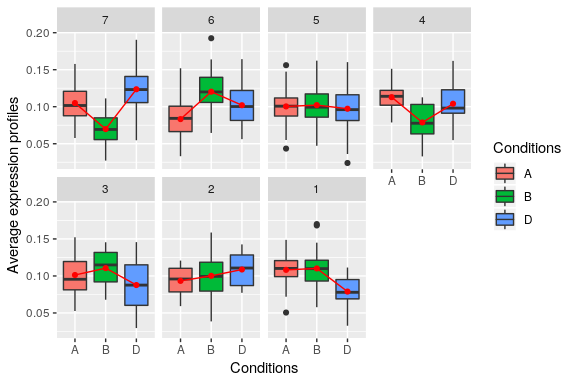We now illustrate an example where we (1) change the theme to black-and-white; (2) set the aspect ratio of y/x to be equal to 20; (3) change the widths of the axis ticks and lines; (4) change the size of the text labels; and (5) change the color scheme. Remember to load the ggplot2 package prior to adding customization! library(ggplot2) p$boxplot +
theme_bw() +
coord_fixed(ratio = 20) +
theme(axis.ticks = element_line(color="black", size=1.5),
axis.line = element_line(color="black", size=1.5),
text = element_text(size = 15)) +
scale_fill_brewer(type = "qual")
## Scale for 'fill' is already present. Adding another scale for 'fill',
## which will replace the existing scale.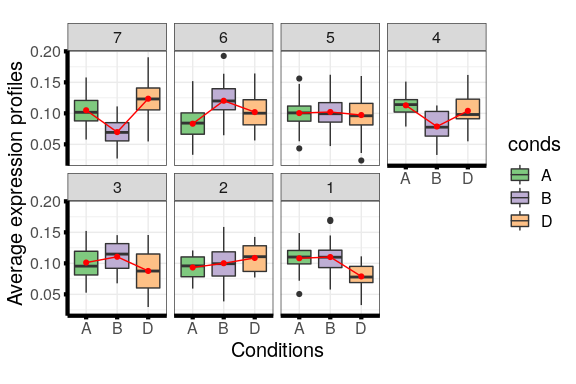## coseq FAQs

1. Should I use the Gaussian mixture model (model="Normal") or the K-means (model="kmeans") approach for my co-expression analysis? And what about the Poisson mixture model?

The Gaussian mixture model is more time-intensive but enables the estimation of per-cluster correlation structures among samples. The K-means algorithm has a much smaller computational cost, at the expense of assuming a diagonal per-cluster covariance structures. Generally speaking, we feel that the K-means approach is a good first approach to use, although this can be somewhat context-dependent. Finally, although we feel the Poisson mixture model was a good first approach for RNA-seq co-expression, we now recommend either the Gaussian mixture model or K-means approach instead.

1. There are a lot of proposed transformations in the package. Which one should I use?

In our experience, when using the Gaussian mixture model approach the arcsine transformation performs well. When using the K-means algorithm, if highly specific profiles should be highlighted (e.g., genes expressed in a single tissue), the logCLR transformation performs well; if on the other hand the user wishes to distinguish the fine differences among genes with generally equal expression across conditions (e.g., non-differentially expressed genes), the CLR transformation performs well.

1. Why do I get different results when I rerun the analysis?

Because the results of coseq depend on the initialization point, results from one run to another may vary; as such, in practice, it is typically a good idea to re-estimate the same collection of models a few times (e.g., 5) to avoid problems linked to initialization.

1. How do I output the clustering results?

Use the clusters(...) command for cluster labels, and the assay(...) command for conditional probabilities of cluster membership.

1. How can I check whether all models converged?

Look at the nbClusterError slot of the coseqResults metadata using metadata(...)\$nbClusterError to examine the models that did not converge.

1. Can I run coseq on normalized profiles that have already been pre-computed?

Yes, you should just specify normFactors = "none" and transformation = "none" when using coseq.

1. Does it matter if I have an unbalanced experimental design?

Condition labels are not used in the actual clustering implemented by coseq, and in fact are only used when visualizing clusters using the plot command if collapse_rows = c("sum", "average"). As such, the clustering itself should not be unduly influenced by unbalanced designs.

1. Why did I get an error message about the singular covariance matrices for the form of Gaussian mixture model I used?

Occasionally, the default form of Gaussian mixture model (Gaussian_pk_Lk_CK, which is the most general form available with per-cluster proportions, volumes, shapes, and orientations for covariance matrices) used in coseq is not estimable (via the Rmixmod package used by coseq) due to non-invertible per-cluster covariance matrices. The error message thus suggests using a slightly more restrictive form of Gaussian mixture model, such as the Gaussian_pk_Lk_Bk (which imposes spherical covariance matrices) or Gaussian_pk_Lk_I (which imposes diagonal covariance matrices). See ?Rmixmod::mixmodGaussianModel for more details about the nomenclature and possible forms of Gaussian mixture models.